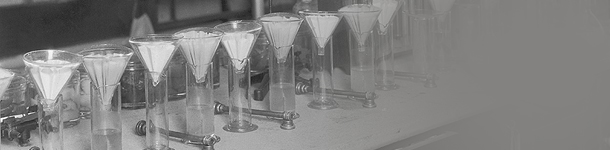• EN /

#### Select Your Language / Currency Language / Currency

##### Currency
Save
• 18620722006
circular dichroism spectroscopy
Circular dichroism (CD) is the most widely used method for the determination of protein secondary structure and is a fast, simple and accurate method for studying protein conformation in dilute solution. It can be measured in solution, closer to its physiological state. Moreover, the determination method is rapid and simple and sensitive to conformational changes. Therefore, it is one of the main methods for studying the secondary structure of proteins and has been widely applied to the conformational studies of proteins.
Introduction
An optical spectrum used to deduce the configuration and conformation of asymmetric molecules. The absorption coefficient (ε) of the optically active substance on the left-handed and right-handed circularly polarized lights constituting plane-polarized light is not equal, and εL ≠ εR, that is, circular dichroism. If the wavelength λ of the plane polarized light with different wavelengths is taken as the abscissa and the difference between the absorption coefficients Δε = εL-εR is taken as the ordinate, the obtained spectrum is the circular dichroism spectrum, or CD for short. If a chiral compound is absorbed in the UV region, a characteristic circular dichroism can be obtained. Since εL ≠ εR, the transmitted light is no longer plane-polarized light, but elliptically polarized light. The relation between the molar ovality [θ] and Δε is: [θ] = 3300Δε. Circular dichroism can also be ellipticity of the Moore for the vertical axis, the wavelength of abscissa for mapping. Circular dichroism also has positive circular dichroism with peaks and negative circular dichroism with valleys due to the positive and negative values ​​of Δε. Circular dichroism and spin spectroscopy were measured in the UV-visible region with the purpose of deducing the configuration and conformation of the organic compound.
principle
Light is transverse electromagnetic waves, is a ray of vibration in all directions. The electric field vector E and the magnetic field vector H are perpendicular to each other and perpendicular to the light wave propagation direction. As the main role of the photosensitive effect of the electric field vector, the electric field vector is generally used as the light wave vibration vector. The plane of light wave electric field vector and direction of propagation is called the vibration surface of light wave. If the vibration surface does not change with time, this beam of light is called plane polarized light, the vibration surface is called the polarization plane. Plane polarized light can be decomposed into two circularly polarized lights of the same amplitude and frequency and opposite rotation directions. The electrical vector, referred to as right-handed circularly polarized light rotating in a clockwise direction, is referred to as left-handed circularly polarized light, rotating in a counterclockwise direction. Two beams of the same amplitude and frequency, the opposite direction of rotation of the polarized light can also be synthesized into a plane of plane polarized light. If the amplitudes (intensities) of the two beams of polarized light are not the same, then a beam of elliptically polarized light will be synthesized. The company is located in:
The optical active material has different absorptivities of left and right circularly polarized light, and the difference in light absorption ΔA (Al - Ad) is called the circular dichroism (abbreviated as CD) of the material. The presence of circular dichroism alters the plane-polarized light propagating through the material into elliptically polarized light and is only visible at wavelengths where absorption occurs. The ellipticity of the formed ellipse θ is: θ = tg-1 Short axis / long axis
According to the Lambert-Beer law, the ellipticity can be approximated as follows: θ = 0. 576 lc (εl - εd) = 0. 576 lcΔε In the formula, l is the medium thickness, c is the concentration of the photoactive substance, εl and εd are the substances Absorption coefficient for left-handed and right-handed circularly polarized light. The relationship between the value of θ (or Δε) at different wavelengths and the wavelength λ, ie the circular dichroism spectral curve, is measured. In this spectral curve, if the measured substance has no characteristic absorption, its Δε value is very small, that is, the characteristic circular dichroism spectrum can not be obtained. When εl> εd, a positive circular dichroism curve is obtained, that is, the measured substance is dextrorotary. If εl <εd, a negative circular dichroism curve is obtained, that is, the measured substance is left-handed. The company is located in:
According to the principle of circular dichroism spectroscopy and test requirements designed instrument called circular dichroism spectrometer. At present, circular dichroism spectrometry and its instruments have been widely used in organic chemistry, biochemistry, coordination chemistry and medicinal chemistry fields, and become an important method to study the three-dimensional configuration of organic compounds.

Products
Analytical instruments
Physical property test
Environmental monitoring and analysis
Lab general equipment